• 图形变换
图形变换
二维几何变换

1.向量基本知识
2.坐标系的分类：世界坐标系，局部坐标系
3.二维图形几何变换：齐次坐标，二维几何变换，物体变换和坐标变换，复合坐标
4.窗口视区及变换：窗口，视区，窗口到视区的变换

三维图形变换

1.三维物体基本几何变换
2.三维物体的投影变换：透视投影，平行投影

以上都是基本的数学知识，涵盖于SLAM，机器人学，线性代数等多个领域。


展开全文• 汇编图形变换，画圆，不同颜色边框的正方形，不同颜色的三角形
• 用MFC开发的简单图形变换程序，主要包括图形的平移、缩放、旋转等等 用MFC开发的简单图形变换程序，主要包括图形的平移、缩放、旋转等等
• 计算机图形学 图形变换 旋转 平移等。。本人花了很多时间编的、、运行通过的。。方便。直接运行。。
• 基本图形变换：平移、旋转、变比、对称。符合变换：相对非原点的变比，绕非原点旋转。 关键字：二维图形变换,平移,旋转,变比
•3d
• OpenGL图形变换OpenGL中的变换模型视图矩阵平移变换旋转变换缩放变换矩阵堆栈使用投影正射投影透视投影裁剪变换 OpenGL中的变换 OpenGL中的常用变换包括视图变换、模型变换、模型视图变换、投影变换和视见区变换。 ...
OpenGL图形变换OpenGL中的变换模型视图矩阵平移变换旋转变换缩放变换矩阵堆栈使用投影正射投影透视投影裁剪变换
OpenGL中的变换
OpenGL中的常用变换包括视图变换、模型变换、模型视图变换、投影变换和视见区变换。
模型视图矩阵
OpenGL提供了一些函数用于把任意矩阵设置成当前操作的矩阵（模型视图矩阵或投影矩阵），例如：
glfloat m[]={ 1.0f, 1.0f, 1.0f, 1.0f,
1.0f, 1.0f, 1.0f, 1.0f,
1.0f, 1.0f, 1.0f, 1.0f,
1.0f, 1.0f, 1.0f, 1.0f, };
glMatrixMode(GL_MODELVIEW);
glLoadMatrixf(m);

平移变换
void glTranslated(GLdouble x, GLdouble y, GLdouble z);
void glTranslatef(GLfloat x, GLfloat y, GLfloat z);
旋转变换
缩放变换
矩阵堆栈
使用投影
正射投影
透视投影
裁剪变换


展开全文OpenGL
• Canvas 图形变换 图形学领域倾向于对于任何图形的绘制都先绘制图形的基本轮廓，再用图形变换的方式把它绘制成需求的大小。 基本的图形变换： 位移translate ( x ,y )：把图像原点位移到( x ,y )的位置 旋转...
Canvas 图形变换

图形学领域倾向于对于任何图形的绘制都先绘制图形的基本轮廓，再用图形变换的方式把它绘制成需求的大小。

基本的图形变换：

位移translate ( x ,y )：把图像原点位移到( x ,y )的位置
旋转rotate ( deg )：旋转deg的度数
缩放scale ( sx ,sy)：在横向进行sx倍的缩放，在纵向进行sy倍的缩放。
scale缩放具有副作用，体现在scale不仅缩放图像大小，而且对于其他数值属性也有影响，比如左上角的坐标属性和图像边框大小等。

注：canvas的图形变换是叠加的

context.fillStyle="red";
context.translate(100,100);
context.fillRect(0,0,400,400);

context.fillStyle="green";
context.translate(300,300);
context.fillRect(0,0,400,400);

上述代码中描绘的第二个正方形的原点将移动到(400,400)的位置。如果要使第二个正方形的原点将移动到(300,300)的位置，可以使用context.save()和context.restore()。
- context.save()：保存当前的图形状态
- context.restore()：和context.save()成对出现，恢复save()保存的所有状态

context.save();
context.fillStyle=”red”;
context.translate(100,100);
context.fillRect(0,0,400,400);
context.restore();

context.save()
context.fillStyle=”green”;
context.translate(300,300);
context.fillRect(0,0,400,400);
context.restore();

可以在context.save()和context.restore()之间任意的绘制图形效果而不会影响到后面的图形绘制。

变换矩阵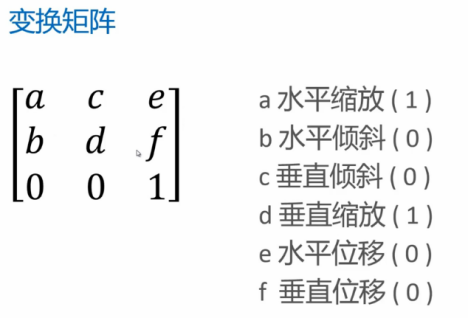在不进行图形变换的情况下，变换矩阵相当于单位矩阵，当我们想对一个图形进行变换的时候，只要对变换矩阵相应的参数进行操作，操作后对图形中各个顶点的坐标分别乘以这个矩阵算出新的顶点坐标即可。
canvas提供了两个设置变换矩阵的方法：
- transform ( a ,b ,c ,d ,e ,f )
- setTransform( a ,b ,c ,d ,e ,f )

transform()和setTransform()的区别：

使用transform()设置变换矩阵，每次设置是在之前的变换矩阵的基础上进行设置，即transform()设置的变换矩阵可以产生级联的关系。
setTransform()首先将当前矩阵设置为单位矩阵，再根据传进来的参数设置矩阵，即setTransform()会忽略掉前面设置的所有transform()。

展开全文• 关于图形变换我们关注的都是怎么得到变换之后的坐标，而对于变换后的坐标，很多教材上都只有一个简略的结果，并不会给出详细的推导过程。今天学习旋转变换的时候，对怎么得出变换后的坐标产生了疑惑，花了几分钟才想...
文章目录齐次坐标[^3]二维旋转变换三维旋转变换绕 z 轴旋转绕 x 轴旋转绕 y 轴旋转总结例子
齐次坐标1
齐次坐标表示法就是用 n+1 维向量表示一个 n 维向量。
n 维空间中的点的位置向量用非齐次坐标表示时，$(P_1,P_2,…, P_n)$ 具有 n 个坐标分量，并且是唯一的。如果用齐次坐标表示时，该向量有 n+1 个坐标分量，$(hP_1, hP_2,…, hP_n, h)$ 并且是不唯一的。2
通常都使 h=1。如果 h≠0，就可以用除齐次坐标的各分量，这一方法称之为齐次坐标的规范化。经过规范化后的齐次坐标就是唯一的。
h = 0 时，该点表示一无穷远点3。三元组 (0, 0, 0) 不表示任何点。原点表示为 (0, 0, 1)
如二维点 (x,y) 的齐次坐标表示为 $(h_x,h_y,h)$ ，则 $(h_1x,h_1y,h_1) ， (h_2x,h_2y,h_2) ，…， (h_mx,h_my,h_m)$ 都是表示二维空间中的同一个点三维空间中的坐标点的齐次坐标可表示为 (x,y,1) 。
应用齐次坐标可以有效地用矩阵运算把二维、三维甚至更高维空间中点集从一个坐标系转换到另一个坐标系中。
二维齐次坐标变换矩阵的形式为
$T_{2D} = \begin{bmatrix} a & d & g \\ b & e & h \\ c & f & i \\ \end{bmatrix}$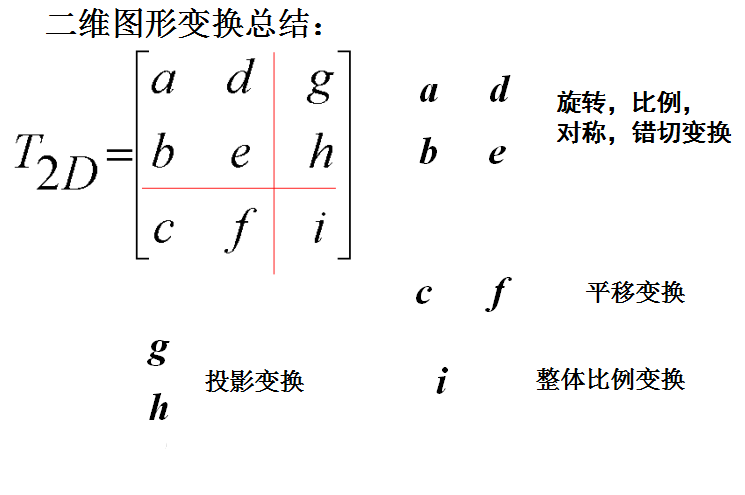三维齐次坐标变换矩阵的形式为
$T_{3D} = \begin{bmatrix} a_{11} & a_{12} & a_{13} & a_{14} \\ a_{21} & a_{22} & a_{23} & a_{24} \\ a_{31} & a_{32} & a_{33} & a_{34} \\ a_{41} & a_{42} & a_{43} & a_{44} \\ \end{bmatrix}$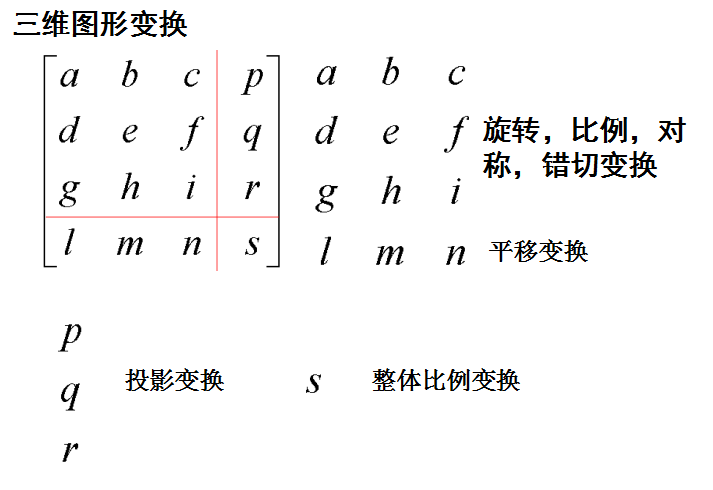二维旋转变换
下面是一个简单的绕原点旋转变换的图。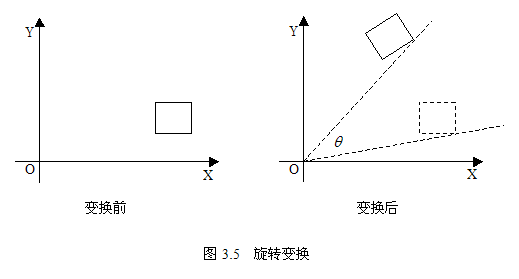本文我们关注的是怎么得到变换之后的坐标，而对于变换后的坐标，很多教材上都只有一个简略的结果，并不会给出详细的推导过程。今天学习旋转变换的时候，对怎么得出变换后的坐标产生了疑惑，花了几分钟才想明白，特此记录一下。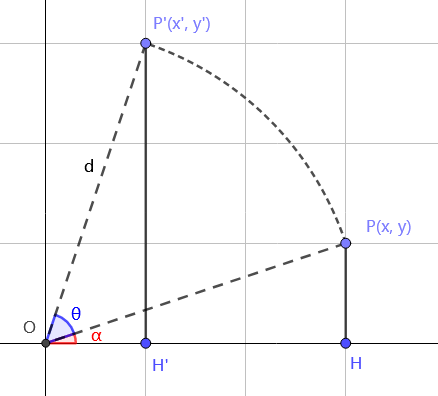我们不妨设变换前图形上任意一点的坐标为 $P(x, y)$，变换后图形上对应点坐标为 $P'(x', y')$ 。
对于旋转变换来说，我们不妨设旋转角度为 θ，即 $\angle POP' = \theta$。我们现在就来推导一下 $x'$ 和 $y'$ 关于 x, y 的表示法。（应该还有更好的证法）
由旋转的性质易知 $OP = OP' = \sqrt{x^2 + y^2} = d$
不妨设 $\angle POx = \alpha$，有 $sin\alpha = \dfrac{y}{d}$， $cos\alpha = \dfrac{x}{d}$。又有下面这两个方程：
$sin(\alpha + \theta) = \dfrac{y'}{d} \newline cos(\alpha + \theta) = \space \space \dfrac{x'}{d}$
把这两个方程解出来就可以得到 x’ 和 y’ 的值了（把 sin(a+θ)，cos(a+θ) 拆开后，sin a 和 cos a 都已知，把 d 乘过去就行，这里打方程不方便就不敲出具体拆解过程了）：
$x' = xcos\theta - ysin\theta \newline y' = xsin\theta + ycos\theta \newline$
因此有如下推导
$P' = [x'\space\space y'\space\space 1]=[xcos\theta - ysin\theta \space\space xsin\theta + ycos\theta \space\space 1]=[x\space\space y \space\space 1]\begin{bmatrix} cos\theta & sin\theta & 0 \\ -sin\theta & cos\theta & 0 \\ 0 & 0 & 1 \end{bmatrix}=P・R(\theta)$4
其坐标变换矩阵 $R(\theta)$ 如下
$\begin{bmatrix} cos\theta & sin\theta & 0 \\ -sin\theta & cos\theta & 0 \\ 0 & 0 & 1 \end{bmatrix}$
三维旋转变换
以下都是往逆时针方向旋转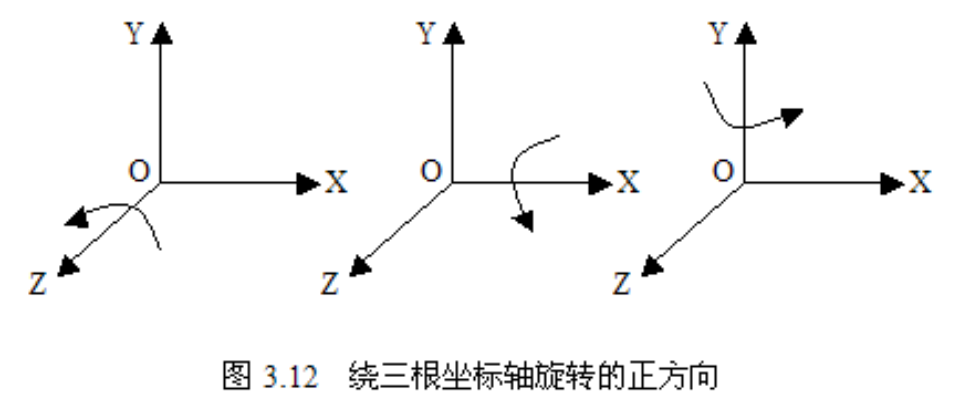分别绕三根坐标轴旋转的时候，有一个坐标是不变的，然后我们把另外两个当成二维的算就行，我们可以分别得出对应的坐标变换矩阵：
绕 z 轴旋转
$\begin{bmatrix} cos\theta & sin\theta & 0 & 0 \\ -sin\theta & cos\theta & 0 & 0 \\ 0 & 0 & 1 & 0 \\ 0 & 0 & 0 & 1 \end{bmatrix}$
绕 x 轴旋转
$\begin{bmatrix} 1 & 0 & 0 & 0 \\ 0 & cos\theta & sin\theta & 0 \\ 0 & -sin\theta & cos\theta & 0 \\ 0 & 0 & 0 & 1 \end{bmatrix}$
绕 y 轴旋转
$\begin{bmatrix} cos\theta & 0 & -sin\theta & 0 \\ 0 & 1 & 0 & 0 \\ sin\theta & 0 & cos\theta & 0 \\ 0 & 0 & 0 & 1 \end{bmatrix}$
如果说要记得话，一个简单的记法是绕哪个轴旋转哪个轴上就没有 cos sin 之类的。
总结
这个总结可以不看，现在我也看不懂了。
我们不难发现核心部分基本和二维都是差不多的，除了绕 y 轴旋转的那个 sinθ 的正负号反了。那么我们有没有想过为什么就它是反的呢？一个歪理如下：因为核心部分$\begin{bmatrix} cos\theta & sin\theta \\ -sin\theta & cos\theta \\ \end{bmatrix}$被分开了 😃以下结论对初始点在两轴正方向成立，对旋转角度也有一定要求：

对于绕 z 轴旋转，观察到 x 一直在减小
对于绕 x 轴旋转，观察到 y 一直在减小
对于绕 y 轴旋转，观察到 z 一直在减小

而坐标变换矩阵中，谁减小，负号就在谁的 sinθ 那里。
例子
例：设三维空间中有一条任意直线，它由直线上一点 Q 和沿直线方向的单位方向向量 n 确定。Q 点坐标为 $(x_0, y_0, z_0)$，直线向量 n = $[n_1, n_2, n_3]$ ，|n| = $\sqrt{{n_1}^2+{n_2}^2+{n_3}^2}$ = 1。求绕这条直线旋转 $\theta$ 角的旋转变换矩阵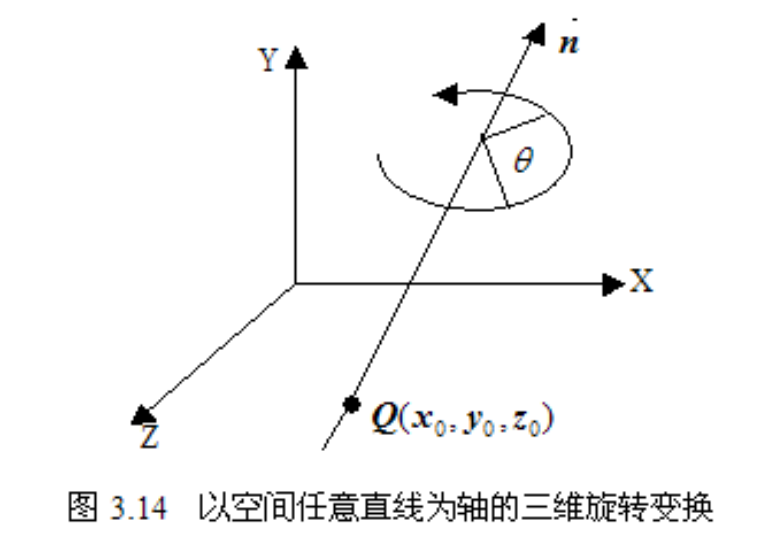实现该变换可以先做平移变换，使旋转轴成为通过坐标原点的一条直线，然后做绕通过坐标原点的旋转轴旋转 $\theta$ 角的旋转变换，最后再做平移变换，即开始所作平移变换的逆变换，使旋转轴平移回到原来的位置。
过坐标原点的任意直线为旋转轴的旋转变换可分为五步实现：

做绕 X 轴旋转 $\alpha$ 角的变换，使旋转轴落在 Y=0 上。
做绕 Y 轴旋转 $\beta$ 角的变换，使旋转轴与 Z 轴重合。
做绕 Z 轴旋转 $\theta$ 角的旋转变换。
做第 2 步的逆变换，即做绕 Y 轴 -$\alpha$ 旋转变换。
做第 1 步的逆变换，即做绕 X 轴 -$\beta$ 旋转变换。

我们不妨假设由向量 ON 表示直线向量 n = $[n_1, n_2, n_3]$，其中 O 为原点，N 坐标为 $(n_1, n_2, n_3)$。对题中直线平移到原点后，绕题中的直线旋转就相当于绕 ON 旋转。
我们要确定的值是 $\alpha$ 和 $\beta$ 这两个角，首先我们来看 $\alpha$
$\alpha$ 是绕 X 轴旋转的变换的角，使平面 OMN 落在 Y=0 上成为 $OM''N''$
$sin\alpha = \dfrac{M'M}{OM} = \dfrac{n_2}{v}$
$cos\alpha = \dfrac{M'O}{OM} = \dfrac{n_3}{v}$
其中 $OM = v = \sqrt{{n_2}^2+{n_3}^2}$，M’ 为 M 到 OZ 的垂足。
由于是绕 X 轴旋转，故其旋转矩阵为
$R_x(\alpha) = \begin{bmatrix} 1 & 0 & 0 & 0 \\ 0 & cos\alpha& sin\alpha& 0 \\ 0 & -sin\alpha& cos\alpha & 0 \\ 0 & 0 & 0 & 1 \end{bmatrix} = \begin{bmatrix} 1 & 0 & 0 & 0 \\ 0 & \dfrac{n_3}{v} & \dfrac{n_2}{v} & 0 \\ 0 & -\dfrac{n_2}{v} & \dfrac{n_3}{v} & 0 \\ 0 & 0 & 0 & 1 \end{bmatrix}$
$N' = [n_1, n_2, n_3, 1] R_x(\alpha) = [n_1, 0, v, 1]$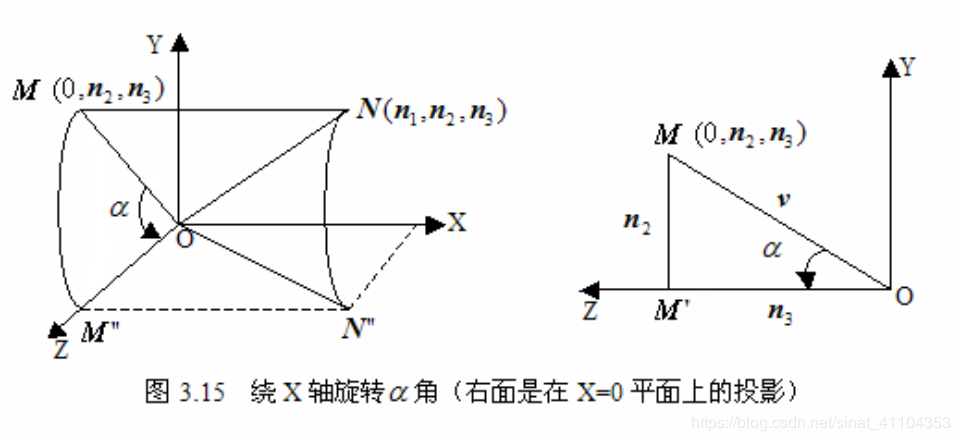然后我们再来看 $\beta$，$\beta$ 是在第一步的基础上绕 Y 轴旋转 $ON''$ 使得 $ON''$ 落在 Z 轴上
由第一步我们已经知道 $M''N'' = MN = n_1$，$d = ON'' = ON = \sqrt{{n_1}^2+{n_2}^2+{n_3}^2} = 1$，所以有
$sin\beta= -\dfrac{M''N''}{ON''} = -\dfrac{n_1}{d}=-n_1$5
$cos\beta= \dfrac{M''O}{ON''} = \dfrac{\sqrt{d^2-{n_1}^2}}{d}=\sqrt{d^2-{n_1}^2}=\sqrt{n_2^2+n_3^2}=v$
由于 $\beta$ 是绕 Y 轴旋转，故其旋转矩阵为
$R_y(\beta) = \begin{bmatrix} cos\beta & 0 & -sin\beta& 0 \\ 0 & 1 & 0 & 0 \\ sin\beta& 0 & cos\beta& 0 \\ 0 & 0 & 0 & 1 \end{bmatrix} = \begin{bmatrix} v & 0 & n_1 & 0 \\ 0 & 1 & 0 & 0 \\ -n_1 & 0 & v & 0 \\ 0 & 0 & 0 & 1 \end{bmatrix}$
$N'' = [n_1, 0, v, 1]R_y(\beta)=[0,0,1,1]$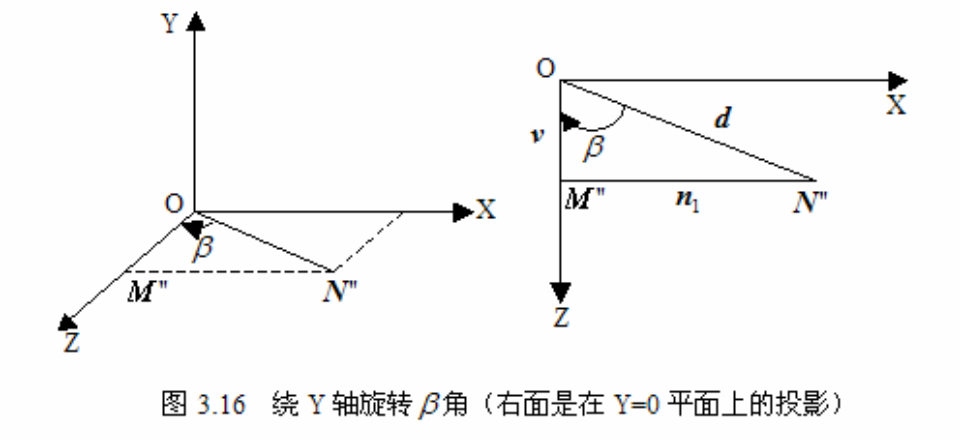又做绕 Z 轴旋转 θ 角的旋转变换。故旋转矩阵为
$R_z(\theta)=\begin{bmatrix} cos\theta & sin\theta & 0 & 0 \\ -sin\theta & cos\theta & 0 & 0 \\ 0 & 0 & 1 & 0 \\ 0 & 0 & 0 & 1 \end{bmatrix}$
回到 5 个步骤

做绕 X 轴旋转 $\alpha$ 角的变换，使旋转轴落在 Y=0 上。
做绕 Y 轴旋转 $\beta$ 角的变换，使旋转轴与 Z 轴重合。
做绕 Z 轴旋转 $\theta$ 角的旋转变换。
做第 2 步的逆变换，即做绕 Y 轴 -$\alpha$ 旋转变换。
做第 1 步的逆变换，即做绕 X 轴 -$\beta$ 旋转变换。

五个步骤用旋转矩阵表示出来如下：
$R(\theta)=R_x(\alpha)・R_y(\beta)・R_z(\theta)・R_y(-\beta) ・R_x(-\alpha) =$
$\begin{bmatrix} 1 & 0 & 0 & 0 \\ 0 & \dfrac{n_3}{v} & \dfrac{n_2}{v} & 0 \\ 0 & -\dfrac{n_2}{v} & \dfrac{n_3}{v} & 0 \\ 0 & 0 & 0 & 1 \end{bmatrix}$ ・$\begin{bmatrix} v & 0 & n_1 & 0 \\ 0 & 1 & 0 & 0 \\ -n_1 & 0 & v & 0 \\ 0 & 0 & 0 & 1 \end{bmatrix}$ ・$\begin{bmatrix} cos\theta & sin\theta & 0 & 0 \\ -sin\theta & cos\theta & 0 & 0 \\ 0 & 0 & 1 & 0 \\ 0 & 0 & 0 & 1 \end{bmatrix}$ ・$\begin{bmatrix} v & 0 & -n_1 & 0 \\ 0 & 1 & 0 & 0 \\ n_1 & 0 & v & 0 \\ 0 & 0 & 0 & 1 \end{bmatrix}$ ・$\begin{bmatrix} 1 & 0 & 0 & 0 \\ 0 & \dfrac{n_3}{v} & -\dfrac{n_2}{v} & 0 \\ 0 & \dfrac{n_2}{v} & \dfrac{n_3}{v} & 0 \\ 0 & 0 & 0 & 1 \end{bmatrix}=$
$\begin{bmatrix} n_1^2+(1-n_1^2)cos\theta & n_1n_2(1-cos\theta)+n_3sin\theta & n_1n_3(1-cos\theta)-n_2sin\theta & 0 \\ n_1n_2(1-cos\theta)-n_3sin\theta & n_2^2+(1-n_2^2)cos\theta & n_2n_3(1-cos\theta)+n_1sin\theta & 0 \\ n_1n_3(1-cos\theta)+n_2sin\theta & n_2n_3(1-cos\theta)-n_1sin\theta & n_3^2+(1-n_3^2)cos\theta & 0 \\ 0 & 0 & 0 & 1 \end{bmatrix}$

齐次坐标可让包括无穷远点的点坐标以有限坐标表示 ↩︎

[计算机图形学 第四版]: 齐次坐标（homogeneous coordinate）这一术语在数学中用来指出笛卡尔方程的表达效果。当笛卡尔点 (x, y) 转换成齐次坐标 $(x_h, y_h, h)$ 时，包含 x 和 y 的方程 f(x, y) = 0 变成了三个参数 $x_h, y_h 和 h$ 的齐次方程。这恰好说明，假如三个参数均被各自乘上值 v 后的值替换，那么 v 可以从方程中作为因子提取出来。 ↩︎

[维基百科，齐次坐标]:  一条通过原点 (0, 0) 的线之方程可写作 nx+my = 0，其中 n 及 m 不能同时为 0。以参数表示，则能写成 x=mt, y=-nt。令 Z=1/t，则线上的点之笛卡尔坐标可写作 (m/Z, -n/Z)。在齐次坐标下，则写成 (m, -n, Z)。当 t 趋向无限大，亦即点远离原点时，Z 会趋近于 0，而该点的齐次坐标则会变成 (m, -n, 0)。因此，可定义 (m, -n, 0) 为对应 nx+my=0 这条线之方向的无穷远点之齐次坐标。因为欧式平面上的每条线都会与透过原点的某一条线平行，且因为平行线会有相同的无穷远点，欧式平面每条线上的无穷远点都有其齐次坐标。 ↩︎

[维基百科，齐次坐标]: 有些作者会使用不同的符号表示齐次坐标，以与笛卡尔坐标相区别，如以冒号代替逗号，如以 (x:y:z) 代替 (x, y, z)，以强调该坐标有着比例的性质。亦有以方括号代替括弧，如以 [x, y, z] 来强调有多个坐标表示同一个点。有些作者则会同时使用冒号与方括号，如 [x:y:z]。 ↩︎

这里 $sin\beta$ 是负的是因为 $\beta$ 是顺时针旋转，我们默认逆时针方向为正方向。 ↩︎


展开全文计算机图形学
• 有极详细的坐标轴变换，画出任意多边形，可选定相对的图形变换方式，图形变换功能比较齐全。
• 图形变换 c++二维图形的基本几何变换，如平移、旋转、缩放、对称、错切变换
• 介绍3dmax的图形变换什么是图形变换图形变换有什么作用？ 有哪些基本的二维（三维）几何变换？ 如何计算组合变换？难点与解决办法。 小结
• 摘要:VC/C++源码,界面编程,图形变换,窗体换肤 有六种风格，二维图形变换演示程序，可以一键切换窗口到XP风格、Office风格Win2000风格和Office2007风格等，在二维图形变换方面，在旋转中心四周按箭头指示方向（切线...
• 完成一个图形变换的程序，系统具有 4 种可选择的功能，按字母“Y”画一个用点组成的圆；按字母“S”画一个用不同颜色填充的三角形；按字母“Z”画一个用不同颜色填充的矩形；按“q”键退出。
• 计算机图形学二维图形变换 计算机图形学二维图形变换 两种方法实现图形变换
• 面向对象的编程文件，基于c++语言创建的图形变换cpp，应用visual studio软件，适用于初学者加强对课程的理解和应用，希望学习资源能够给您带来帮助！c++
• OpenGL图形变换编程实例，实现了OpenGL封装的关于图形变换的函数
• 图形变换矩阵     | 包括平面的和空间的 如果把点定义为行向量，那么根据矩阵的乘法，变换矩阵的形式只能是这样的： P'=PT 其中的P’...
• 探讨了基于Visual Basic的图形变换处理方法．首先简要介绍了PaintPicture，AlphaBlend和PlgBlt等函数的功能，然后具体讨论了几种典型的图形变换算法的实现过程，最后指出通过这些方法，借助特定的数学模型，ViSual ...
• 计算机图形学中的图形变换方法。实用方便。对图形进行处理。变换。...